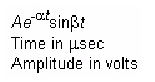GraphicsUsing Character and Numeric Variables in Text

Any string variable is a valid specification for the text String property. This section illustrates how to use matrix, cell array, and numeric variables as arguments to the text function.

Character Variables

For example, each row of the matrix PersonalData contains specific information about a person (note that all but the longest row are padded with a space so that each has the same number of columns).

PersonalData = ['Jack Straw  ';'489 Main St.';'Wichita KN '];

To display the data, index into the desired row.

• text(x1,y1,['Name: ',PersonalData(1,:)])
text(x3,y3,['City and State: ',PersonalData(3,:)])


Cell Arrays

Using a cell array enables you to create multiline text with a single text object. Each cell does not need to be the same number of characters. For example, the following statements,

• key(1)={'{\itAe}^{-\alpha\itt}sin\beta{\itt}'};
key(2)={'Time in \musec'};
key(3)={'Amplitude in volts'};
text(x,y,key)


produce this output.Numeric Variables

You can specify numeric variables in text strings using the num2str (number to string) function. For example, if you type on the command line

• x = 21;
['Today is the ',num2str(x),'st day.']


MATLAB concatenates the three separate strings into one.

• Today is the 21st day.


Since the result is a valid string, you can specify it as a value for the text String property.

• text(xcoord,ycoord,['Today is the ',num2str(x),'st day.'])Mathematical Symbols, Greek Letters, and TEX Characters Example -- Multiline Text